# Math Insight

### Elementary derivative problems

#### Problem 1

Below is a graph of the function $h(p)$.

1. For what values of $p$ is $\diff{h}{p}$
1. negative?
2. positive?
3. zero?
4. undefined?
2. Find all critical points of $h$.
3. For what values of $p$ is $\diffn{h}{p}{2}$
1. negative?
2. positive?
3. zero?
4. undefined?
4. Find all inflection points of $h$.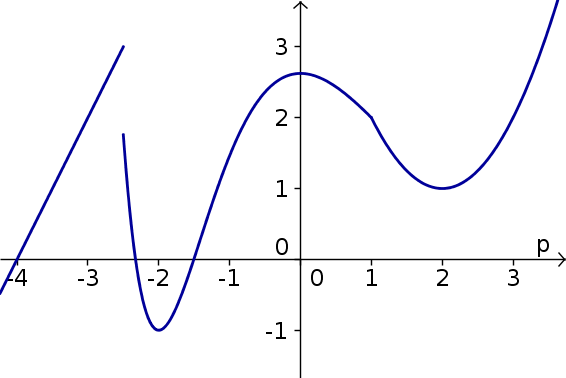#### Problem 2

Below is a graph of the function $r(z)$.

1. For what values of $z$ is $r'(z)$
1. negative?
2. positive?
3. zero?
4. undefined?
2. Find all critical points of $r$.
3. For what values of $z$ is $r''(z)$
1. negative?
2. positive?
3. zero?
4. undefined?
4. Find all inflection points of $r$.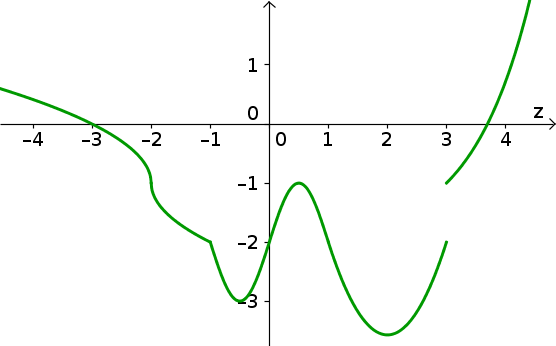#### Problem 3

Let $f(b)$ be the function graphed below. Identify critical points, inflection points, and locations where the derivative and second derivative of $f$ are positive and negative. Use these results to graph $f'(b)$.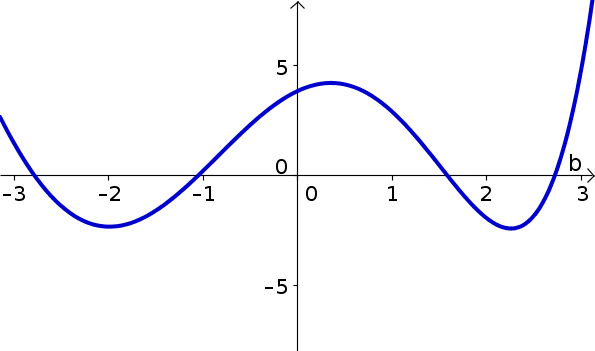#### Problem 4

Let $w(y)$ be the function graphed below. Identify critical points, inflection points, and locations where the derivative and second derivative of $w$ are positive and negative. Use these results to graph $\diff{w}{y}$.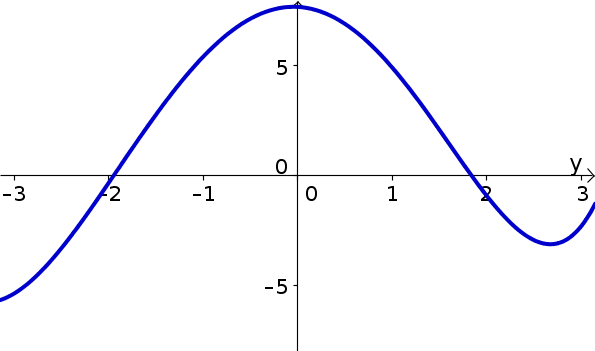#### Problem 5

Let $u(x)$ be the function graphed below. Identify critical points, inflection points, and locations where the derivative and second derivative of $u$ are positive and negative. Use these results to graph $\diff{u}{x}$ and $\diffn{u}{x}{2}$.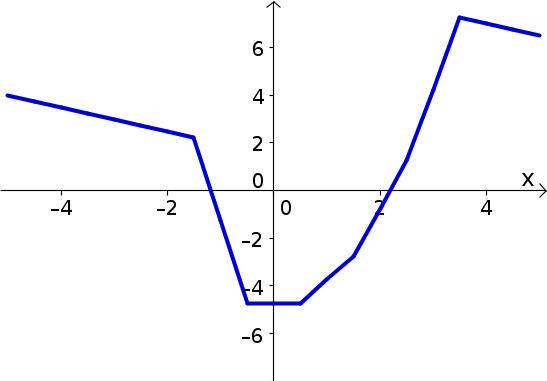#### Problem 6

Let $g(z)=5z^2-z+22$. Find a linear function that is a good approximation of $g$ for values of $z$ near $3$.

#### Problem 7

Let $g(z)=5z^2-z+22$. Find the equation for the tangent line to the graph of $g$ at the point $z=3$.

#### Problem 8

Let $k(q)=qe^{-q}$. Find the linear approximation for $k$ around $q=a$.

#### Problem 9

Let $m(y)=e^{y^2}$. Find the equation for the tangent line of the graph of $m$ at $y=b$.

#### Problem 10

Let $x(t) = t^3 \ln (t)$. What is the slope of the tangent line to the graph of $x$ at the point $t=2$. At the point $t=\bigstar$?

#### Problem 11

Let $y(z)=\ln(az)$ for some positive parameter $a$.

1. What is $\diff{y}{z}$?
2. What is $\diffn{y}{z}{2}$?

#### Problem 12

Let $z(y)=ce^{by}$ for some parameters $b$ and $c$.

1. What is $z'(y)$?
2. What is $z'(0)$?
3. What is $z'(1/b)$?
4. What is $z''(y)$?
5. What is $z''(0)$?
6. What is $z''(1/b)$?

#### Problem 13

Let $h(s)=(a^2+b^2)e^{s^2}$ for parameters $a$ and $b$.

1. What is $h'(s)$?
2. What is $h'(1)$?
3. What is $h''(s)$?
4. What is $h''(1)$?

#### Problem 14

Let $s(u)=\frac{1+u}{1-u}$. What is $s'(u)$?

#### Problem 15

Let $g(x)=x^2e^{-x}$. Find $g'(x)$.

#### Problem 16

Let $f(x)=x^ne^{-x}$, where $n$ is a parameter. Find $f'(x)$.

One you have worked on a few problems, you can compare your solutions to the ones we came up with.